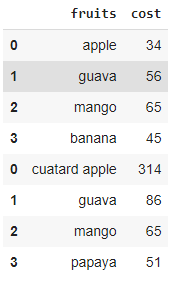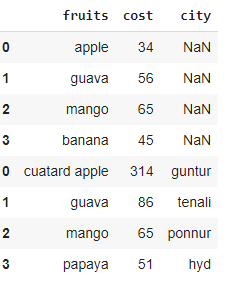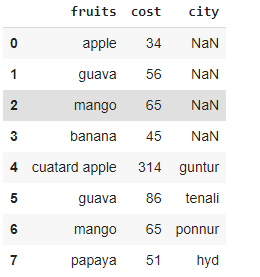# How to Use rbind in Python?

## Method 1: Use rbind() function with equal columns

Here we have to take 2 dataframes with equal columns and apply concat() function. This will combine the rows based on columns.

Syntax:

`pandas.concat([dataframe1, dataframe2])`

where

• dataframe1 is the first dataframe
• dataframe2 is the second dataframe

Example:

## Python3

 `# import pandas module ` `import` `pandas as pd ` ` `  `# create first dataframe ` `data1 ``=` `pd.DataFrame({``'fruits'``: [``'apple'``, ``'guava'``, ``'mango'``, ``'banana'``], ` `                      ``'cost'``: [``34``, ``56``, ``65``, ``45``]}) ` ` `  `# create second dataframe ` `data2 ``=` `pd.DataFrame({``'fruits'``: [``'cuatard apple'``, ``'guava'``, ``'mango'``, ``'papaya'``], ` `                      ``'cost'``: [``314``, ``86``, ``65``, ``51``]}) ` ` `  `# concat two columns ` `pd.concat([data1, data2]) `

Output:## Method 2: Use rbind() function with unequal columns

Here the two dataframes columns are not equal, In this scenario, the unmatched column will get NAN replaced rows in its column.

Syntax:

`pandas.concat([dataframe1, dataframe2])`

where,

• dataframe1 is the first dataframe
• dataframe2 is the second dataframe

Example:

## Python3

 `# import pandas module ` `import` `pandas as pd ` ` `  `# create first dataframe with 2 columns ` `data1 ``=` `pd.DataFrame({``'fruits'``: [``'apple'``, ``'guava'``, ``'mango'``, ``'banana'``], ` `                      ``'cost'``: [``34``, ``56``, ``65``, ``45``]}) ` ` `  `# create second dataframe with 3 columns ` `data2 ``=` `pd.DataFrame({``'fruits'``: [``'cuatard apple'``, ``'guava'``, ``'mango'``, ``'papaya'``], ` `                      ``'cost'``: [``314``, ``86``, ``65``, ``51``], ` `                      ``'city'``: [``'guntur'``, ``'tenali'``, ``'ponnur'``, ``'hyd'``]}) ` ` `  `# concat two columns ` `pd.concat([data1, data2]) `

Output:Here we observed that the index of the rows again starts from 0, in order to avoid this, we have to use the .reset_index() method. This will reset the index of the new dataframe.

Syntax:

`pandas.concat([dataframe1, dataframe2]).reset_index(drop=True)`

Example:

## Python3

 `# import pandas module ` `import` `pandas as pd ` ` `  `# create first dataframe with 2 columns ` `data1 ``=` `pd.DataFrame({``'fruits'``: [``'apple'``, ``'guava'``, ``'mango'``, ``'banana'``], ` `                      ``'cost'``: [``34``, ``56``, ``65``, ``45``]}) ` ` `  `# create second dataframe with 3 columns ` `data2 ``=` `pd.DataFrame({``'fruits'``: [``'cuatard apple'``, ``'guava'``, ``'mango'``, ``'papaya'``], ` `                      ``'cost'``: [``314``, ``86``, ``65``, ``51``], ` `                      ``'city'``: [``'guntur'``, ``'tenali'``, ``'ponnur'``, ``'hyd'``]}) ` ` `  `# concat two columns ` `pd.concat([data1, data2]).reset_index(drop``=``True``) `

Output:Whether you're preparing for your first job interview or aiming to upskill in this ever-evolving tech landscape, GeeksforGeeks Courses are your key to success. We provide top-quality content at affordable prices, all geared towards accelerating your growth in a time-bound manner. Join the millions we've already empowered, and we're here to do the same for you. Don't miss out - check it out now!

Previous
Next